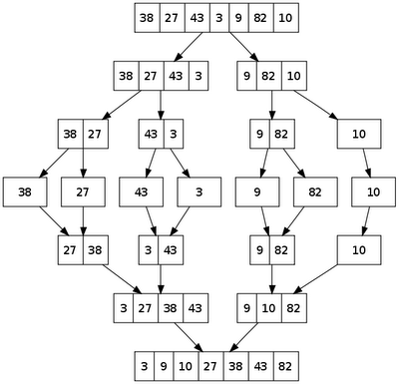# Jef Claes

On software and life

The latest addition to my [data structures and algorithms in JavaScript](https://github.com/JefClaes Data-structures-and-algorithms-in-JavaScript) is the merge sort algorithm.

There are four main steps in the merge sort algorithm (from Wikipedia):

• If the list is of length 0 or 1, then it is already sorted.

Otherwise:

• Divide the unsorted list into two sublists of about half the size.
• Sort each sublist recursively by re-applying the merge sort.
• Merge the two sublists back into one sorted list.

I found it a lot easier to understand the algorithm by just looking at this diagram (also from Wikipedia).I added a public `mergeSort` function to the `sortArray` object, which I showed in the first post in these series.

``````this.mergeSort = function() {
elements = internalMergeSort(elements, this.onSort);
return elements;
};
``````

This function calls the `internalMergeSort` function passing in the internal elements array and the onSort callback.

``````var internalMergeSort = function(elements, onSort){
if (elements.length < 2){
return elements;
}

// Calculate the middle of the elements
var middle = Math.floor(elements.length / 2);
// Divide
var leftRange = elements.slice(0, middle);
var rightRange = elements.slice(middle, elements.length);
// Conquer
var mergingResult = merge(internalMergeSort(leftRange, onSort),
internalMergeSort(rightRange, onSort));
onSort(mergingResult);

return mergingResult;
};
``````

The function recursively divides the elements in two parts, sorting them and finally merging them back together.

The `merge` function is implemented like this.

``````
function merge(left, right){
var res = [];

while (left.length > 0 && right.length > 0) {
if (left <= right) {
res.push(left.shift());
} else {
res.push(right.shift());
}
}

while (left.length > 0) {
res.push(left.shift());
}

while (right.length > 0) {
res.push(right.shift());
}

return res;
};
``````

I peeked at this implementation, which is very similar to mine and which helped me write the first while loop more elegantly.

Testing sorting algorithms is pretty easy. So far, I have only defined one QUnit test for this algorithm.

``````test("MergeSort sorts.", function() {
var sortArray = new algorithms.sortArray();

sortArray.push(15);
...
sortArray.push(130);

var actual = sortArray.mergeSort();
var expected = [1, 10, 12, 15, 17, 22, 25, 50, 60, 90, 130];

deepEqual(actual, expected);
});
``````

A small remark here is that you should use deepEqual instead of `equal` for array assertions. We want to compare the contents of the array, not their references.